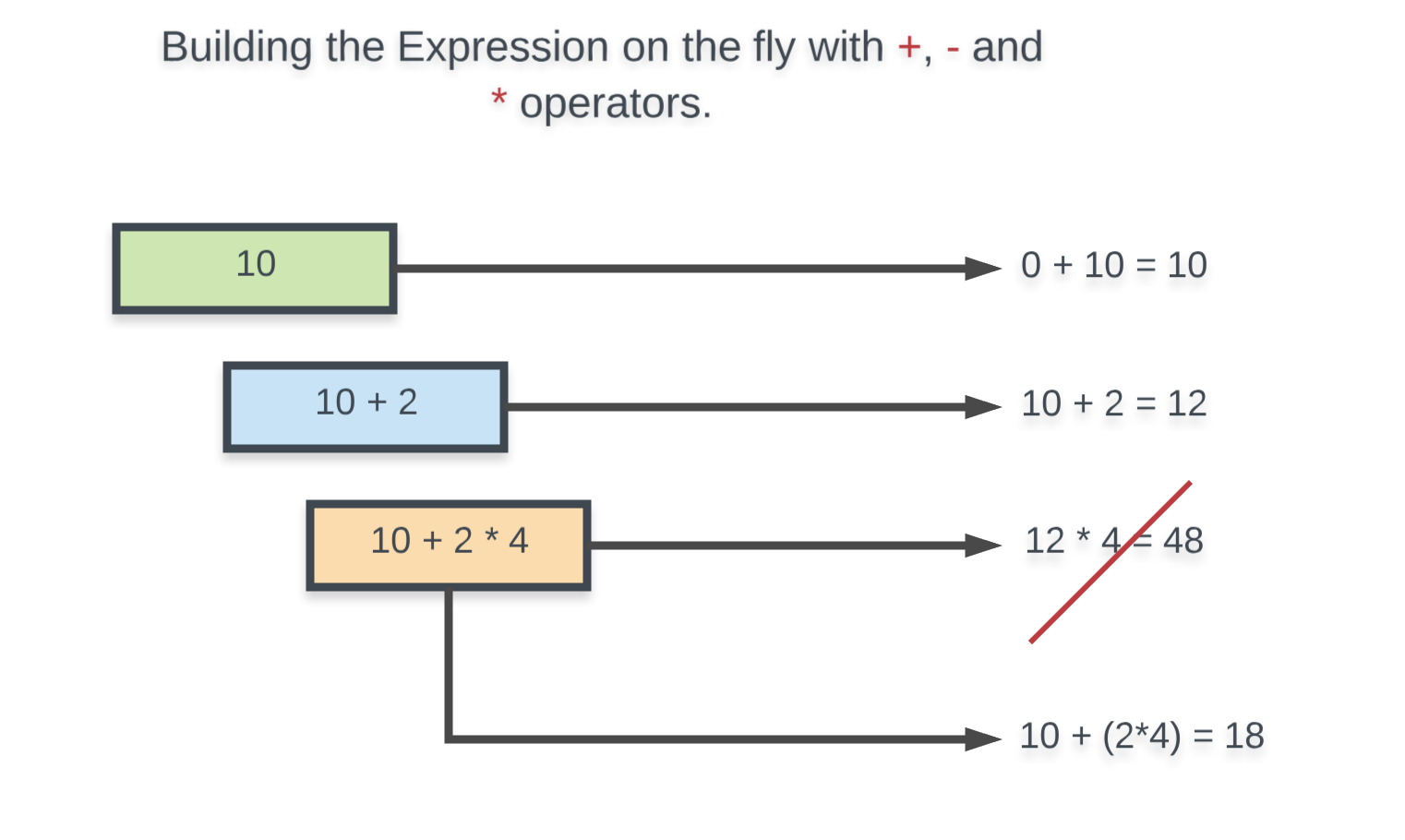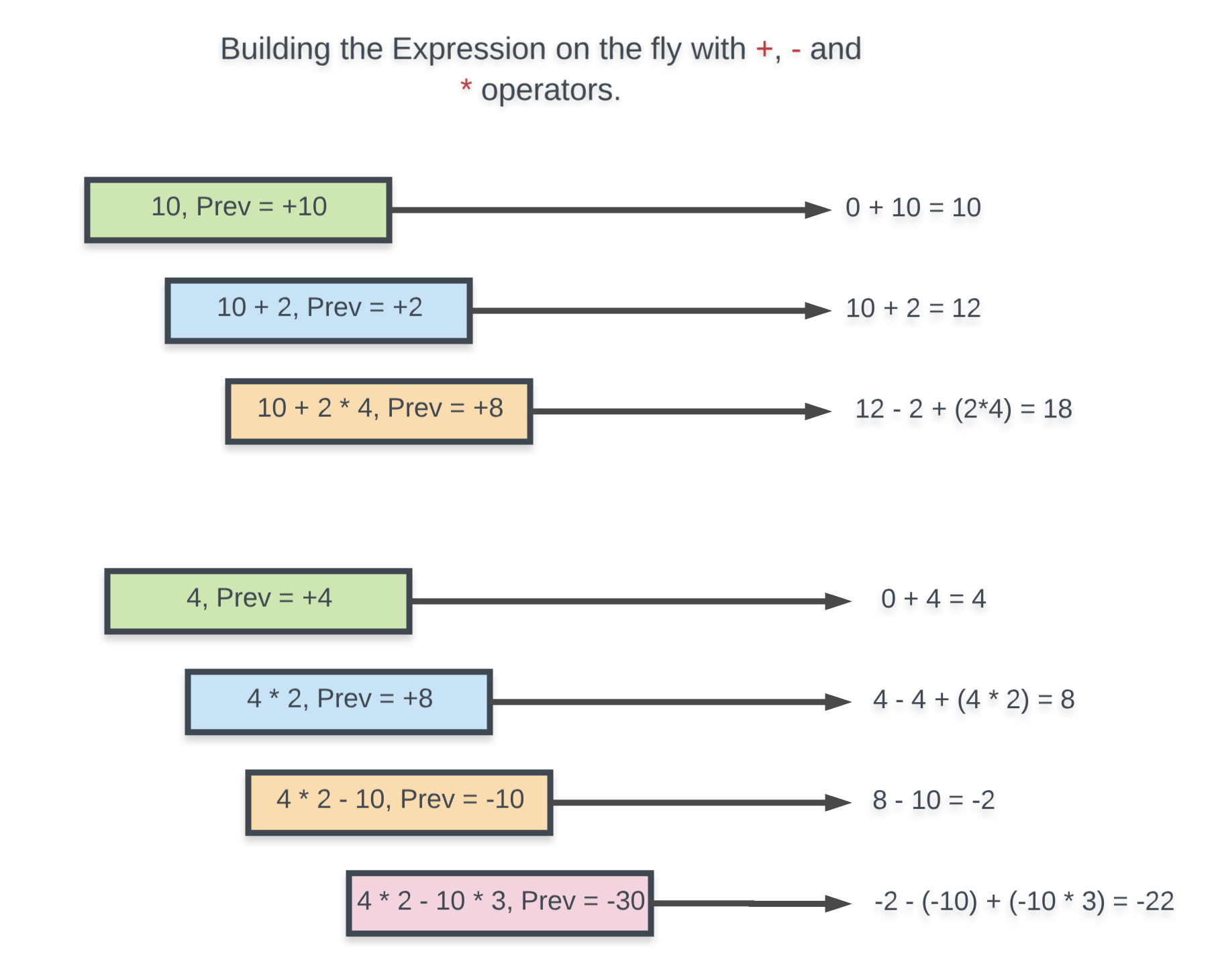## Solution

#### Approach 1: Backtracking

Intuition

Let us first look at what the question asks us to do before getting at the approach to solve it. So, we are given a string of numbers and 3 different operators:

• + Addition,
• - Subtraction or
• * Multiplication

We have to find all possible combinations of binary operators between the digits so that the overall value of the resulting expression becomes equal to a given target value. Let us look at a few possibilities of what it means exactly to place the operators between digits so that the question becomes clearer.

Let's say we are given the following set of digits "123456789" and the target value given to us is 45. Let us see some of the possible resulting expressions that we can get by placing the operators in different locations.

1 + 2 + 3 + 4 + 5 + 6 + 7 + 8 + 9 = 45
1 + 2 - 3 + 4 - 5 + 6 - 7 + 8 - 9 = -3
1 + 2 * 3 - 4 + 5 + 6 - 7 * 8 - 9 = -51
1 + 2 + 3 + 4 + 5 - 6 * 7 + 8 * 9 = 45


These are just 4 of the many resulting expressions that are possible by using the given string of digits and the three operators.

By looking at the above examples we can't really figure out any specific pattern among the resulting expressions that tells us which of them will give us the resulting target.

Since the question explicitly states that we are given binary operators, this means that each of the operator would require two operands.

We can consider each of our digits as an operand.

This means that between every pair of digits we can have any of the three operators i.e. , or .

If you've looked at the question's statement and the examples that are given in the question, you would realize that there is an example where the digits are "105" and the target value is 5. For this particular example, there are two expressions given to us and they are 1*0+5 and 10-5.

The second expression is something that you need to look out for before getting to solve this question because this complicates things a bit.

It would have been an easier question to solve if we just had to consider those expressions that simply had digits as operands.

But, in this question, we can have all sorts of digits getting together and forming a bigger number that becomes a part of the expression. Let us look at some example expressions for the digits "123456" and target 30.

1 * 23 - 4 + 5 + 6 = 30
12 - 3 * 4 + 5 * 6 = 30
1 - 23 - 4 + 56 = 30


So this means that although the number of operators are defined for us i.e. 3 different binary operators, but the number of operands are not really well defined for us.

This is a big portion of the original problem that we need to address in our solution.

Since we are asked to find out all of the valid expressions whose value equals the given target and we don't really know what specific operator between two operands would eventually give us a valid expression,

We try out all of the options.

This means once we have defined what the operands are for our given expression, we would have three possible choices of operators between each consecutive pair of operands.

From an implementation perspective, what would an operand imply with respect to our original string?

An operand would be an integer formed from a substring of our original string.

Let's look at two different array partitions for the given string "123456789"Since we are required to return all of the valid expressions that evaluate to a given target value, we have to try all possible partitions of the given array thereby considering all of the possible operands that can be formed from the digits.

There is a very simple way of incorporating this into our algorithm. Right now, at every point in the algorithm, we have three different choices corresponding to the three different operators.

The way we incorporate these partitions is by considering a 4th operator as well which simply moves one step forward and extends the current operand by one digit. Essentially, going from 12 --> 123 is a NO OP operand in our implementation. (12 * 10) + 3.

Now we have 4 different recursion paths in our algorithm and we have to try out all of them to see which ones lead to a potential solution.

This try out everything hints at a backtracking solution and that is exactly what we are going to look at here.

Algorithm

Let's quickly look at the steps involved in our backtracking algorithm before looking at the pseudo-code.

1. As discussed above, we have multiple choices of what operators to use and what the operands can be and hence, we have to look at all the possibilities to find all valid expressions.
2. Our recursive call will have an index which represents the current digit we're looking at in the original nums string and also the expression string built till now.
3. At every step, we have exactly 4 different recursive calls. The NO OP call simply extends the current_operand by the current digit and moves ahead. Rest of the recursive calls correspond to +, -, and *.
4. We keep on building our expression like this and eventually, the entire nums string would be processed. At that time we check if the expression we built till now is a valid expression or not and we record it if it is a valid one.
1. procedure recurse(digits, index, expression):
2.     if we have reached the end of the string:
3.         if the expression evaluates to the target:
4.             Valid Expression found!
5.     else:
6.         try out operator 'NO OP' and recurse
7.         try out operator * and recurse
8.         try out operator + and recurse
9.         try out operator - and recurse


The algorithm now looks pretty straightforward. However, the implementation is something that needs more thought and there are some things that we need to address before actually looking at the implementation.

When we are done building an expression out of all of the digits in our original string i.e. the base case, then we check if the expression is a valid expression or not. Right ?

How do we actually check if an expression is a valid one or not if all we have is a string representing the expression and not the integer value for the same?

Well, one way to go about this is to write a custom eval function that takes in a string and returns the value of that expression. If you do that (Python people can use the inbuilt function eval for this), you will get a TLE i.e. time limit exceeded error.

Can't we keep track of the expression's value on the fly?

Well yes. That's the idea we will go with. Instead of just keeping track of what the expression string is, we will also keep track of it's value along the way so that when the recursion hits the base case, we can check in time if the expression's value equals the target value or not.

The implementation would have been straightforward had it just been + and - operators involved. This is because both these operators have an equal precedence. That means that we can continue to evaluate the expression on the fly without any problems. Have a look at the following example.So far so good. Now let us add the * operator as well and see how building the expression on the fly like this breaks.What we mean by building the expression on the fly is that we keep track of the expression's value till now and we simply consider that value as one of the two operands for our operators. As we can see from the two examples above, this would have worked had it just been + and - operators.

But, this approach is bound to fail because the * operator takes precedence over + and -. The * operator would require the actual previous operand in our expression rather than the current value of the expression. i.e. In the above example, the * operator needed 2 rather than 12 to get us the correct value of 18.

How to handle this?

The idea on how to handle this problem springs from the discussion above. We simply need to keep track of the last operand in our expression and how it modified the expression's value overall so that when we consider the * operator, we can reverse the effects of the previous operand and consider it for multiplication. Let's take a look at the example that was breaking before.Now we can look at the actual implementation of this algorithm.

Complexity Analysis

• Time Complexity:

• At every step along the way, we consider exactly 4 different choices or 4 different recursive paths. The base case is when the value of index reaches i.e. the length of the nums array. Hence, our complexity would be .
• For the base case we use a StringBuilder::toString operation in Java and .join() operation in Python and that takes time. Here represents the length of our expression. In the worst case, each digit would be an operand and we would have digits and operators. So . This is for one expression. In the worst case, we can have valid expressions.
• Overall time complexity = .
• Space Complexity:

• For both Python and Java implementations we have a list data structure that we update on the fly and only for valid expressions do we create a new string and add to our answers array. So, the space occupied by the intermediate list would be since in the worst case the expression would be built out of all the digits as operands.
• Additionally, the space used up by the recursion stack would also be since the size of recursion stack is determined by the value of index and it goes from all the way to .
• We don't consider the space occupied by the answers array since that is a part of the question's requirement and we can't reduce that in any way

EDIT: The previous implementation of the algorithm, although correct, lead me to write an incorrect complexity analysis section. I've re-written the algorithm from scratch and corrected the complexity analysis as well. Sorry for the inconvenience to all the readers. The core idea of the algorithm is still the same. That hasn't changed.

Special thanks to @ufarooqi, @vortexwolf for providing correct complexity analysis in the discussion forum leading to corrections in the article. Pardon me if I've missed out on any other names :)